# Cosinoidal plane wave hitting a linear medium box

## Cosinoidal plane wave hitting a linear medium box

An arbitrary number of linear medium shapes can be placed inside the Maxwell simulation box.

Linear media are considered a separate system type, for example:


%Systems
'Maxwell' | maxwell
'Medium'  | linear_medium
%


The box shape can be defined in two ways, which are given by the variable LinearMediumBoxShape: if set to medium_parallelepiped, the parallelepiped box will be defined by its center, and size in each dimension through the LinearMediumBoxSize block; if the box shape is defined as medium_box_file, the box shape will be read from an external OFF file, defined through the variable LinearMediumBoxFile. To produce such files, check the Creating geometries tutorial. In either case, the electromagnetic properties of each medium must be defined in the LinearMediumProperties block, which specifies the relative electric permittivity, relative magnetic permeability, and the electric and magnetic conductivities (for lossy media). Finally, the spatial profile assumed to calculate gradients at the box edges is defined through the LinearMediumEdgeProfile variable, and can be either “edged” or “smooth” (for linear media shape read from file, it can only be edged).


LinearMediumBoxShape = medium_parallelepiped
%LinearMediumBoxSize
0.0 | 0.0 | 0.0 | 10.1 | 10.1 | 10.1
%
%LinearMediumProperties
5.0 | 1.0 | 0.0 | 0.0
%
LinearMediumEdgeProfile = edged


Note that we also need to change the variables describing the propagation.


# ----- Time step variables -----------------------------------------------------------------------

Maxwell.TDSystemPropagator = exp_mid

timestep = 1 / ( sqrt(c^2/dx_mx^2 + c^2/dx_mx^2 + c^2/dx_mx^2) )
Maxwell.TDTimeStep                = timestep
Medium.TDTimeStep                 = timestep/2
TDPropagationTime                 = 150*timestep


### No absorbing boundaries

click for complete input

To illustrate a laser pulse hitting a linear medium box, in addition to adding the linear_medium system, we need to switch the Maxwell Hamiltonian operator from the 3x3 vacuum representation to the 6x6 coupled representation with the six component Riemann-Silberstein vector that includes also the complex conjugate Riemann-Silberstein vector.


# ----- Maxwell calculation variables -------------------------------------------------------------



# ----- Maxwell box variables ---------------------------------------------------------------------

# free maxwell box limit of 10.0 plus 2.0 for the incident wave boundaries with
# der_order times dx_mx (here: der_order = 4)

lsize_mx = 12.0
dx_mx    = 0.5

Maxwell.BoxShape = parallelepiped

%Maxwell.Lsize
lsize_mx | lsize_mx | lsize_mx
%

%Maxwell.Spacing
dx_mx | dx_mx | dx_mx
%


For this run, we use the previous incident plane wave propagating only in the x-direction but place a medium box inside the Simulation box.


# ----- Maxwell field variables -------------------------------------------------------------------

# laser propagates in x direction

lambda1 = 10.0
omega1  = 2 * pi * c / lambda1
k1_x    = omega1 / c
E1_z    = 0.05
pw1     = 10.0
ps1_x   = - 25.0

%MaxwellIncidentWaves
plane_wave_mx_function | 0 | 0 | E1_z | "plane_waves_function_1"
%

%MaxwellFunctions
"plane_waves_function_1" | mxf_cosinoidal_wave | k1_x | 0    | 0 | ps1_x | 0     | 0 | pw1
%

gnuplot script

Contour plot of the electric field in z-direction after 50 time steps for t=0.11 and 100 time steps for t=0.21: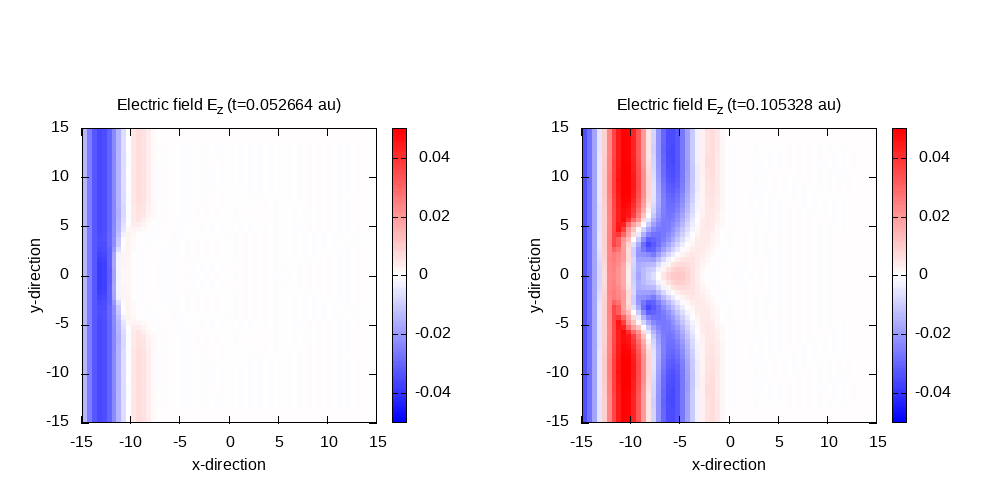Contour plot of the electric field in z-direction after 125 time steps for t=0.26 and 150 time steps for t=0.32: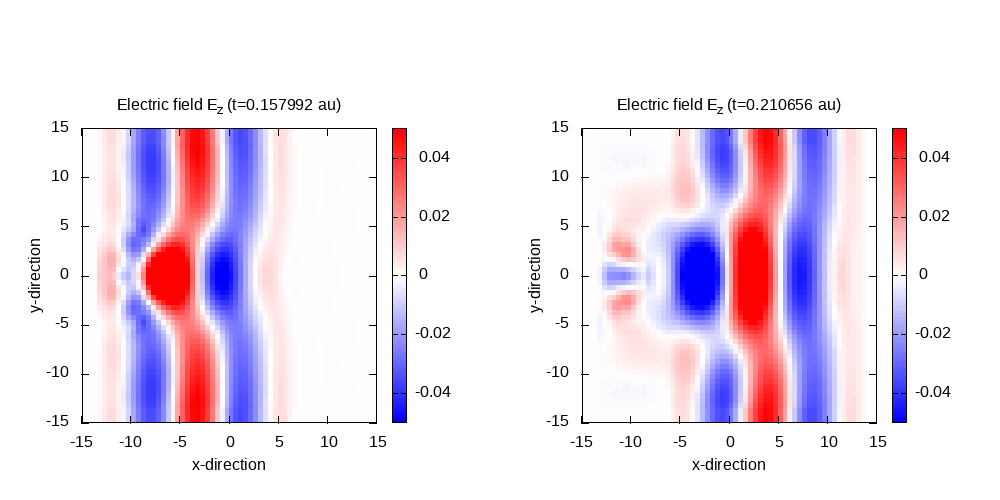In the last panel, it can be seen that there is a significant amount of scattered waves which, in large parts, are scattered from the box boundaries. In the following we will use different boundary conditions, in order to reduce this spurious scattering.

### Mask absorbing boundaries

click for complete input

The previous run was without absorbing boundaries, now we switch on the mask function that damps the field at the boundary by multiplying a scalar mask function. The MaxwellAbsorbingBoundaries block is updated to run with mask absorbing. The MaxwellABMaskWidth is set to five.



# ----- Maxwell boundary conditions -----------------------------------------------------------
%MaxwellAbsorbingBoundaries
%

MaxwellABWidth = 5.0


As a consequence of the additional region for the absorbing boundary condition, we have to change the box size to still obtain the same free Maxwell propagation box. Therefore, the lsize_mx value is now 17.0, which is the previously used size that includes the incident wave boundary width plus the absorbing boundary width. For more details, see the information on simulation boxes


# ----- Maxwell box variables ---------------------------------------------------------------------

# free maxwell box limit of 10.0 plus 2.0 for the incident wave boundaries with
# der_order times dx_mx (here: der_order = 4) plus 5.0 for absorbing boundary conditions

lsize_mx = 17.0
dx_mx    = 0.5

Maxwell.BoxShape = parallelepiped

%Maxwell.Lsize
lsize_mx | lsize_mx | lsize_mx
%

%Maxwell.Spacing
dx_mx | dx_mx | dx_mx
%


Contour plot of the electric field in z-direction after 50 time steps for t=0.11 and 100 time steps for t=0.21: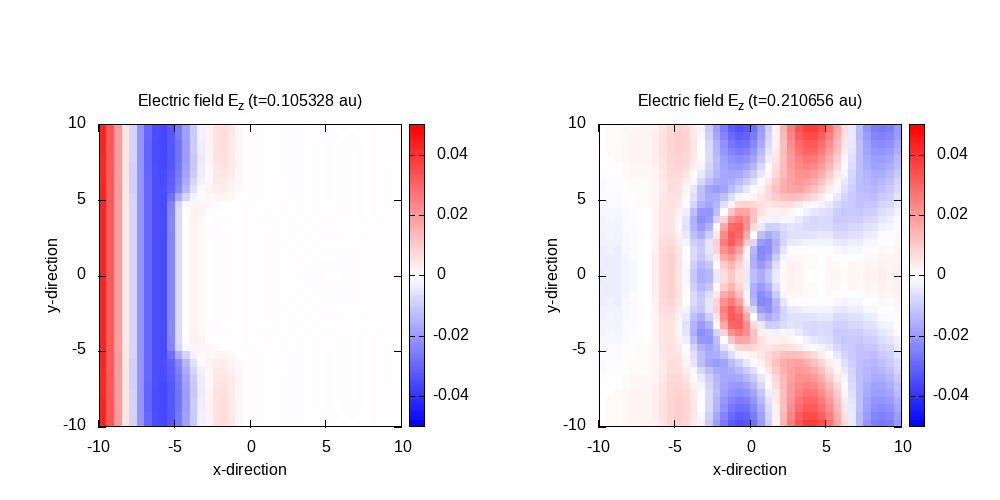Contour plot of the electric field in z-direction after 125 time steps for t=0.26 and 150 time steps for t=0.32: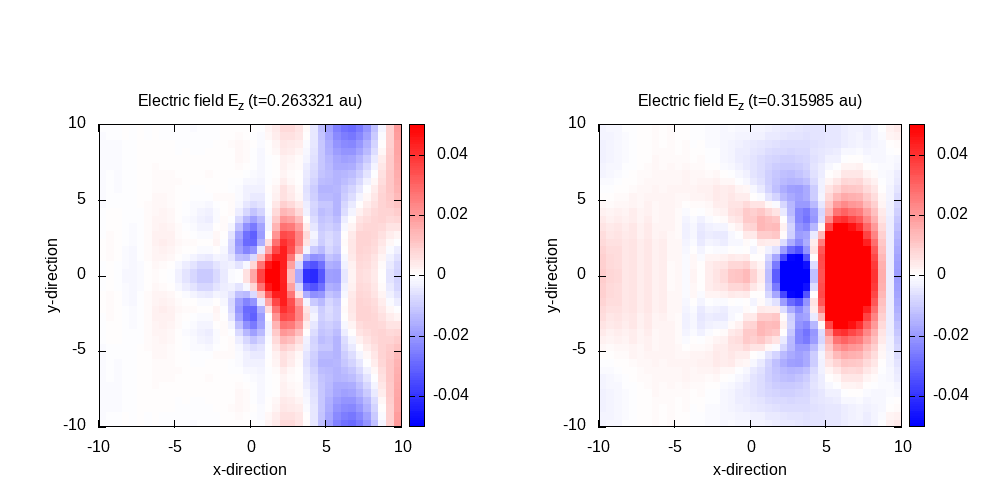It can be seen that the scattering is slightly reduced, but still noticeable.

### Perfectly matched layer boundaries

click for complete input

The mask absorbing method can be replaced by the more accurate perfectly matched layer (PML) method. Therefore, the MaxwellAbsorbingBoundaries by the cpml option. The PML requires some additional parameters to the width for a full definition.



# ----- Maxwell boundary conditions -----------------------------------------------------------
%MaxwellAbsorbingBoundaries
cpml | cpml | cpml
%

MaxwellABWidth = 5.0
MaxwellABPMLPower              = 2.0
MaxwellABPMLReflectionError    = 1e-16


Contour plot of the electric field in z-direction after 50 time steps for t=0.11 and 100 time steps for t=0.21: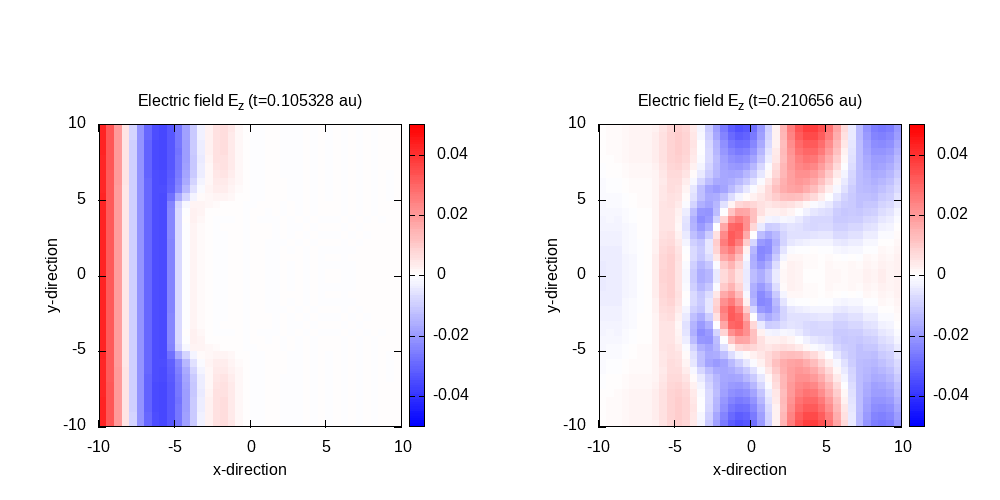Contour plot of the electric field in z-direction after 125 time steps for t=0.26 and 150 time steps for t=0.32: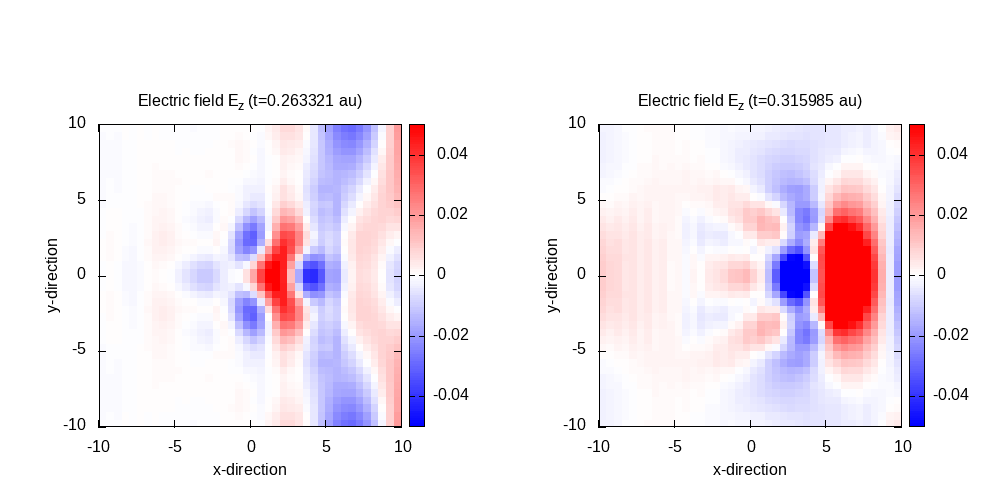The PML boundary conditions in this case are comparable to the mask boundary conditions, but lead to an increased computational cost.